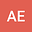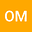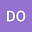Fractal model of a Brownian polymer network: investigation of its dynamic fractal response using an ancient Chinese algorithm
•••• +1Oscar Martínez-Romero
Tecnologico de Monterrey
Author ProfileDaniel Olvera-Trejo
Tecnologico de Monterrey
Author ProfileLuis Palacios-Pineda
Tecnologico Nacional de Mexico
Author Profile## Abstract

In this paper, a mathematical model that describes polymer chain dynamics considering the Brownian motion of particles, an external driving force, the material fractal structure, and a finite extensibility nonlinear elastic (FENE) spring is investigated. To solve the fractal differential equation of motion, first, a two-scale dimension transform is introduced. Then, an ancient Chinese algorithm is used to derive the approximate steady-state frequency-amplitude response curves. Theoretical predictions show that the response shift from the 2D frequency-amplitude curves for fractal parameter values different from one, which modifies the polymer network oscillation amplitudes.